Disjoint Sets (& Why I Have a Favourite Data Structure)
May 3, 2020

Say you have a collection of elements, and you want to group them into a number of sets (that are each disjoint). One example could be grouping nodes within a graph that are connected by a path of edges.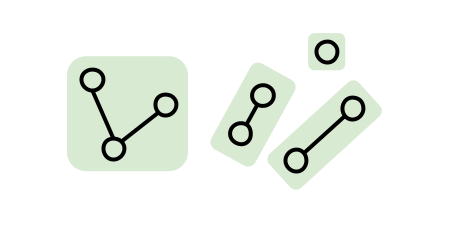There are a number of different data structures you could use to do this, but one which I find particularly elegant is a disjoint set.

• Yes, the plurality is weird here. I tend to just go with “disjoint set” and not think too much about it.
• Yes, I do have a favourite data structure. So should you!

# Basic Operations

Our data structure supports three operations:

• `insert(element)`: Inserts `element`, putting it in its own set. You may see this called “`makeSet`” elsewhere.
• `union(lhs, rhs)`: Union the two sets containing `lhs` and `rhs` (which should have been previously inserted).
• `sameSet(lhs, rhs) -> Bool`: Determine whether `lhs` and `rhs` (which should have been previously inserted) are in the same set. You may see other implementations have a “`findSet`” method instead, that returns a “representative object” for the set containing an element (which can be compared with another to see if two elements are in the same set). I prefer going with `sameSet` directly.

Each set will be represented internally as its own tree. With this representation, the three operations are pretty simple to implement.

# `insert(element)`

• Create a new tree for `element`.# `union(lhs, rhs)`

• Take the root for the tree containing `lhs`.
• Take the root for the tree containing `rhs`.
• If different, move one root to be a child of the other.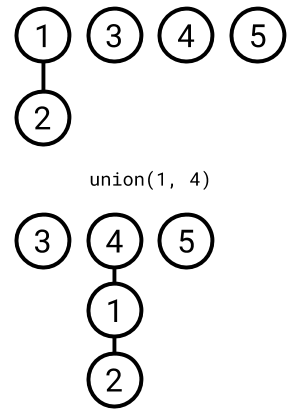# `sameSet(lhs, rhs) -> Bool`

• Take the root for the tree containing `lhs`.
• Take the root for the tree containing `rhs`.
• Return `true` if they’re the same, otherwise `false`.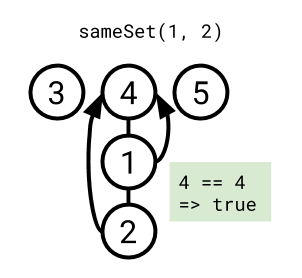# Improvements

The above is all we need to get something working, but there are two heuristics that we can use to improve performance.

• Union by rank: When performing `union`, if one tree is shorter than the other, then move that one. This makes sense if you think about it; we want to try and reduce the length of the path we may need to travel when finding the root of a tree, so we want our trees to be as short as possible.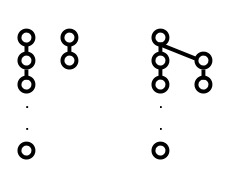The right tree is much shorter than the left, so root it under the left tree on union.
• Path compression: When travelling from a node up to the root of its tree (during a `union`, or `sameSet` operation), move the nodes in the path to point at the root. This way, the next time you need to travel the path, there’s only be a single hop to go for each node. The rationale here is similar to that for union by rank: we want our trees to be as short as possible.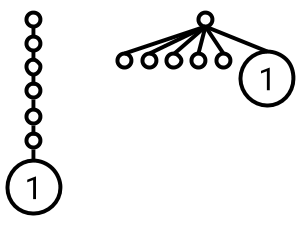After performing an operation such as sameSet(4, other), all nodes on the path from 4 to the root are moved directly under the root. This makes the paths shorter next time around.

# Time Complexity

(Here comes the punchline.)

With those two heuristics in place, it can be shown (using a proof that I admittedly don’t understand) that any sequence of `m` `insert`, `union` and `sameSet` operations can be performed in `O(mα(n))` time (where `n` is the number of inserted elements). `α(n)` is the inverse of the Ackermann function, which grows so slowly that `α(<number of atoms in the universe>) < 5`, so this term is effectively a constant.

Therefore, the amortized complexity of each operation is `O(1)`; each operation is effectively performed in constant time.

How cool is that? I love how such a simple data structure with a few optimizations results in a time complexity based on a function so weird as the Ackermann function.

# Example Implementation

An example implementation in Swift is below (or in a Gist, if you prefer). I’ve tried to thoroughly document the implementation but feel free to reach out if something doesn’t make sense.

``````/// Maintains a number of sets, each containing a number of
/// `Element`s.
///
/// Supports union-ing two sets (using an `Element` contained in
/// each set), as well as determining whether two `Element`s are
/// contained within the same set.
///
/// Note that this is a reference type, rather than a struct
/// with value semantics. This is due to the complexity of
/// copying  the `Node`s on write, which whilst possible,
/// detracts from  the actual behaviour of the type in this
/// example.
public final class DisjointSet<Element: Hashable> {

// MARK: - Types

/// `Error` thrown when an operation expects than an element
/// has already been inserted with `insert(_:)`.
public struct MissingElementError: Error {}

/// A single node in a set.
///
/// Note that this is a reference type, since each `Node` will
/// be stored in the `nodes` dictionary (to lookup `Node`s by
/// an `Element`), and will be referred to by other `Node`s in
/// its set.
private final class Node {
/// The parent `Node`.
///
/// Will be `nil` if this `Node` is the  root node of a set.
var parent: Node?

/// An upper-bound on the number of child nodes of this
/// `Node`.
var rank: Int = 0
}

// MARK: - Properties

/// A mapping of `Element`s to `Node`s, containing a `Node`
/// for each `Element` that has been inserted by `insert(_:)`.
private var nodes: [Element: Node] = [:]

// MARK: - Initializers

/// Initializes a `DisjointSet` that initially contains 0
/// elements.
public init() {}

// MARK: - Public

/// Inserts the specified `element`.
///
/// - Returns: `true` if `element` was inserted, or `false`
///   otherwise (e.g. if `element` has already been inserted).
_ element: Element
) -> Bool {
guard nodes[element] == nil else {
return false
}
nodes[element] = Node()
return true
}

/// Unions the two sets containing `lhs` and `rhs`.
///
/// - Parameters:
///   - lhs: An element contained in the first set.
///   - rhs: An element contained in the second set.
/// - Throws: A `MissingElementError` if either `lhs` or `rhs`
///   has not previously been inserted.
public func union(_ lhs: Element, _ rhs: Element) throws {
guard
let lhsNode = nodes[lhs],
let rhsNode = nodes[rhs]
else {
throw MissingElementError()
}
let lhsRoot = findRoot(node: lhsNode)
let rhsRoot = findRoot(node: rhsNode)
guard lhsRoot !== rhsRoot else {
// `lhs` and `rhs` are already in the same set.
return
}

// Union by rank heuristic.
if lhsRoot.rank > rhsRoot.rank {
// Note: `lhsRoot.rank` is intentionally not incremented
// here. `lhsRoot.rank` is still valid, since it is just
// an upper bound (and the new child, `rhsRoot`, has a
// lower rank).
rhsRoot.parent = lhsRoot
} else if lhsRoot.rank < rhsRoot.rank {
// Note: `rhsRoot.rank` is intentionally not incremented
// here (same reasoning as above).
lhsRoot.parent = rhsRoot
} else {
// `lhsRoot.rank == rhsRoot.rank`. Use `rhsRoot` as the
// new root arbitrarily.
lhsRoot.parent = rhsRoot
// `rhsRoot.rank` needs to be updated here to ensure that
// the upper bound is still valid.
rhsRoot.rank += 1
}
}

/// Returns `true` if `lhs` and `rhs` are members of the same
/// set.
///
/// - Parameters:
///   - lhs: The first `Element` to check for co-membership.
///   - rhs: The second `Element` to check for co-membership.
/// - Throws: `true` if `lhs` and `rhs` are contained in the
///   same set (as a result of a `union(_:_:)` operation.
/// - Returns: A `MissingElementError` if either `lhs` or
///   `rhs` has not previously been inserted.
public func sameSet(
_ lhs: Element,
_ rhs: Element
) throws -> Bool {
guard
let lhsNode = nodes[lhs],
let rhsNode = nodes[rhs]
else {
throw MissingElementError()
}
/// Use `===` to check for instance equality, rather than
/// value equality.
return findRoot(node: lhsNode) === findRoot(node: rhsNode)
}

// MARK: - Private

/// Returns the root `Node` pointed to be `node`, or `node`
/// itself if it is a root node.
private func findRoot(node: Node) -> Node {
if let parent = node.parent {
// Path compression heuristic.
node.parent = findRoot(node: parent)
}
// If `node.parent` is `nil`, `node` is a root node.